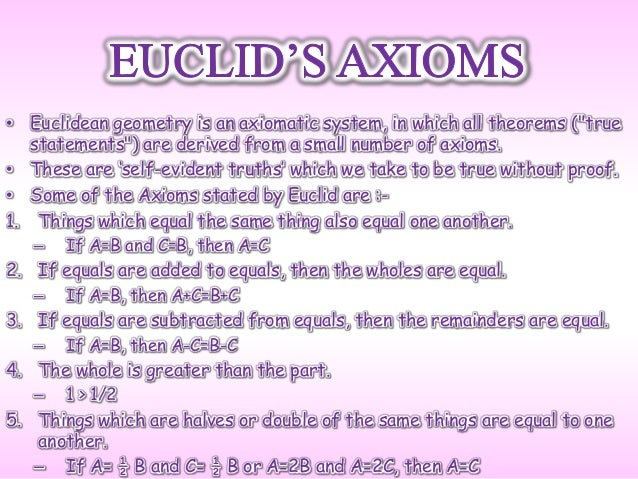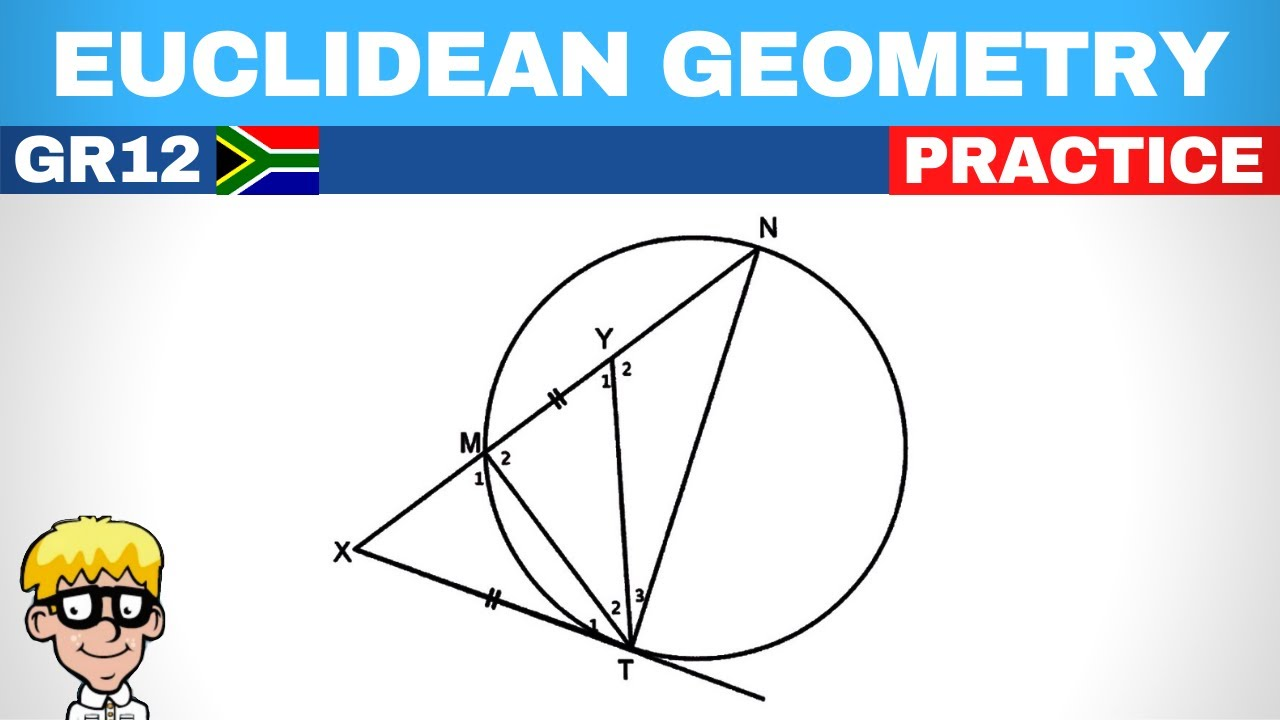# Euclid Geometry Presentation

Introduction to Euclid Geometry
Euclid geometry is a branch of mathematics that was developed by the ancient Greek mathematician Euclid.

It is based on a set of axioms, definitions, and postulates that form the foundation for geometric reasoning.

Euclid's work, particularly his book "Elements," has had a profound influence on mathematics and is still studied today.
Image Source1

Euclid's Axioms and Definitions
Euclid's axioms are self-evident truths or statements that are taken as starting points for reasoning in geometry.

Euclid's definitions provide clear and precise meanings for geometric terms like point, line, and plane.

These axioms and definitions help establish a logical framework for proving geometric theorems.
Image Source2

Euclid's Postulates and Theorems
Euclid's postulates are statements about geometric properties that are accepted without proof.

Theorems in Euclid geometry are proven statements that follow logically from the postulates and axioms.

Euclid's book "Elements" contains hundreds of theorems, covering topics such as triangles, circles, and angles.
Image Source3

Euclidean Geometry in Practice
Euclidean geometry is still widely used today in various fields such as architecture, engineering, and design.

It provides a reliable framework for measuring distances, calculating areas, and solving geometric problems.

Euclid's geometry has also been extended and generalized into non-Euclidean geometries, exploring different geometric systems.
Image Source4

Conclusion
Euclid geometry is a fundamental branch of mathematics that provides the foundation for geometric reasoning.

Euclid's axioms, definitions, postulates, and theorems form the basis of his book "Elements."

The principles and concepts of Euclid geometry continue to be relevant and applicable in various fields of study.
Image Source5